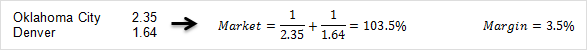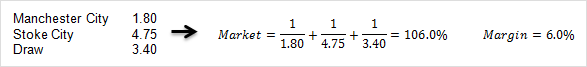Switch ⁄⁄

# BETTING INFORMATION

We offer our visitors relevant and helpful betting articles designed to make them more educated bettors. Through the links you can gain quick access to a wealth of information that will make the world of online betting even more enjoyable.

## AuthorDescription

Australia Sports Betting provides tools, data, news and other resources for sports betting enthusiasts.

Bookmaker, Betting Information

## Bookmaker Margins

User Rating:
(1 ratings)
Aussportsbetting.com
Views: 1214

When a bookmaker sets the odds for a market they first estimate the probability of each possible outcome. For example, in a market on a coin toss winner, the bookmaker will assume the probability of Team X winning is 50%. When using decimal odds, the fair odds for the wager equal the reciprocal of the bookmaker's estimated probability, in this case 1 / 0.50 = 2.00. To fund their operation, the bookmaker adjusts these odds downward to create a profit margin for the market. For example, typical bookmaker odds on Team X winning a coin toss are 1.91. The difference between the published odds (1.91) and the fair odds (2.00) is the bookmaker margin.

The bookmaker margin is a measure of the bookmaker's profit margin for an event and is a hidden transaction cost for punters. This profit is how bookmakers finance their services but bookmakers vary in the margins they apply. From a punter's perspective, the lower the margin, the better. The difference between 1.90 and 1.92 line odds may not seem significant for a single wager, but when betting repeatedly this difference has a compounding effect. This can easily make the difference between winning and losing money.

Below are calculation examples using two-outcome and three-outcome events.Interpreting Bookmaker Margins

The margin measures the bookmaker's profit if they were to receive wagers on each outcome in proportion to the odds. Suppose a bookmaker offers decimal odds a on outcome A and odds b on outcome B. If proportion b/(a+b) is wagered on outcome A and a/(a+b) is wagered on outcome B, then the bookmaker will receive the same profit regardless of the result.

For example, recall that the bookmaker margin for the two-outcome event above was 3.5%. Suppose \$100,000 in total is wagered on the market, with:

(1.64/(1.64 + 2.35)) x \$100,000 = \$41,102.76 wagered on Oklahoma City and
(2.35/(1.64 + 2.35)) x \$100,000 = \$58,897.24 wagered on Denver.

Depending on the outcome, the bookmaker will pay out one of the following two amounts:

If Oklahoma City wins: \$41,102.75 x 2.35 = \$96,591.48
If Denver wins: \$58,897.24 x 1.64 = \$96,591.48

The bookmaker accepts \$100,000 in wagers but only pays out \$96,591.48 to the winners. The profit margin is (\$100,000 - \$96,591.48)/\$96,591.48 = 3.5%, as calculated by the margin earlier.

A bookmaker will rarely receive wagers that are perfectly in proportion to the odds, but over a countless number of events the bookmaker margin will equate to the average profit margin across all events.

Was the article useful?